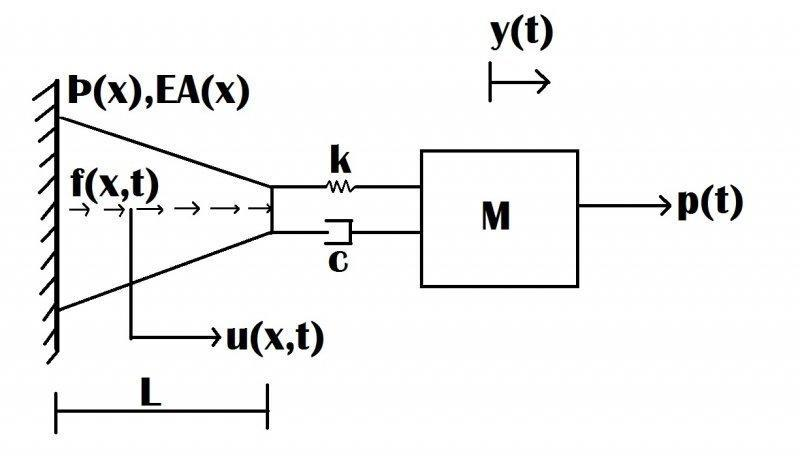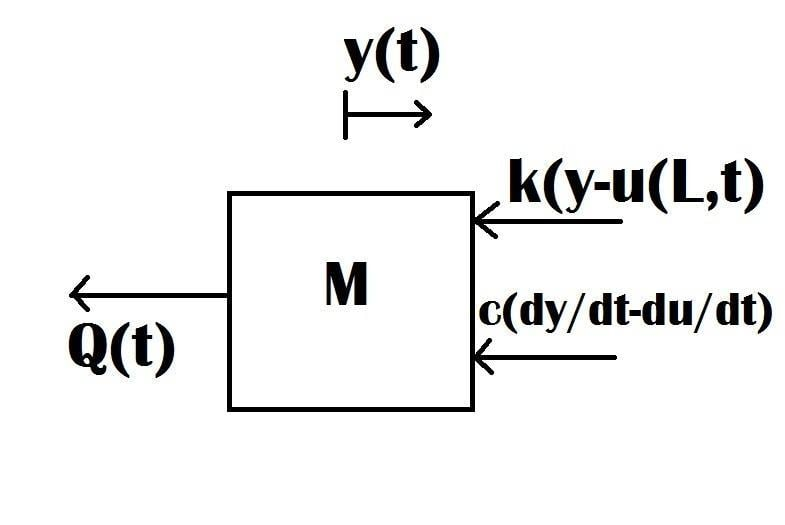# FBD of Flexible and Rigid Body Coupling

ThLiOp
Problem:

I am having a bit of problem with the signs on the force balance with the constraining force. The system is:Attempt:

I drew the FBD with the constraining force for the lumped mass:Then I tried to solve for the constraint force in terms of the spring and damper:For the constraint force, Q(t), I end up getting:

Q(t) = k[u(L,t) - y] + c[(du/dt) - (dy/dt)]

The answer that the professor gives has the signs changed on the k and c terms. Where am I going wrong?

Thank you!

#### Attachments

•